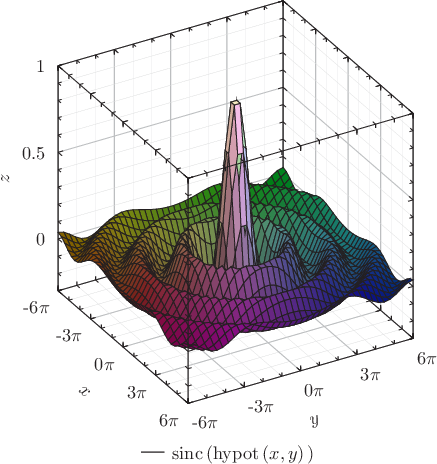# Pyxplot

## Examples - Sinc functionDownload this example:

An example of the surface plotting style

Pyxplot's surface plotting style evaluates a function at a grid of points in the x-y plane, and draws a 3D surface showing how the function varies across the plane. For added prettiness, an expression is also given for the color of the line, which varies from point to point. As in expressions passed to the using modifier, the columns of data are referred to as $1 for the first column, i.e. x;$2 for the second column, y; etc. The expression given here uses the built-in function hsb() to produce a color object with the specified hue, saturation and brightness.

### Script

set numerics complex
set xlabel r"$x$"
set ylabel r"$y$"
set zlabel r"$z$"
set xformat r"%s$\pi$"%(x/pi)
set yformat r"%s$\pi$"%(y/pi)
set xtics 3*pi ; set mxtics pi
set ytics 3*pi ; set mytics pi
set ztics
set key below
set size 6 square
set grid
plot 3d [-6*pi:6*pi][-6*pi:6*pi][-0.3:1] sinc(hypot(x,y)) \
with surface col black \
fillcol hsb(atan2($1,$2)/(2*pi)+0.5,hypot($1,$2)/30+0.2,\$3*0.5+0.5)